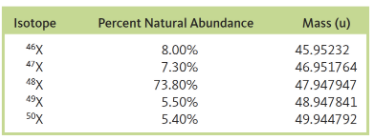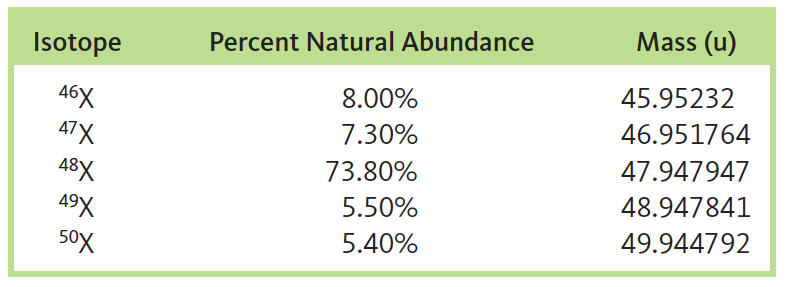# Problem: An element “X” has five major isotopes, which are listed below along with their abundances. What is the element?

###### FREE Expert Solution

We’re being asked to identify the element  using the abundances and masses of its isotopes.

We can use the following equation to calculate the atomic mass:

where:

atomic mass = average atomic mass of the element

f.a. = fractional abundance of the isotope

To get f.a., we simply need to divide the given percent abundance by 100.

We’re given the following values:93% (124 ratings)###### Problem Details

An element “X” has five major isotopes, which are listed below along with their abundances. What is the element?Frequently Asked Questions

What scientific concept do you need to know in order to solve this problem?

Our tutors have indicated that to solve this problem you will need to apply the Atomic Mass concept. You can view video lessons to learn Atomic Mass. Or if you need more Atomic Mass practice, you can also practice Atomic Mass practice problems.

What professor is this problem relevant for?

Based on our data, we think this problem is relevant for Professor Oliver's class at UCSC.

What textbook is this problem found in?

Our data indicates that this problem or a close variation was asked in Chemistry: An Atoms First Approach - Zumdahl Atoms 1st 2nd Edition. You can also practice Chemistry: An Atoms First Approach - Zumdahl Atoms 1st 2nd Edition practice problems.